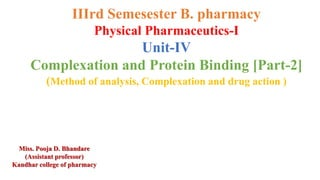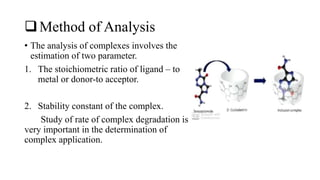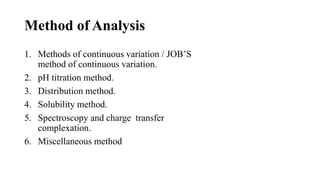Diese Präsentation wurde erfolgreich gemeldet.

# Complexation and Protein Binding [Part-2] (Method of analysis, Complexation and drug action )

Anzeige
Anzeige
Anzeige
Anzeige
Anzeige
Anzeige
Anzeige
Anzeige
Anzeige
Anzeige
Anzeige×

1 von 17 Anzeige

# Complexation and Protein Binding [Part-2] (Method of analysis, Complexation and drug action )

Method of Analysis: Methods of continuous variation / JOB’S method of continuous variation.
pH titration method.
Distribution method.
Solubility method.
Spectroscopy and charge transfer complexation.
Miscellaneous method

Method of Analysis: Methods of continuous variation / JOB’S method of continuous variation.
pH titration method.
Distribution method.
Solubility method.
Spectroscopy and charge transfer complexation.
Miscellaneous method

Anzeige
Anzeige

### Complexation and Protein Binding [Part-2] (Method of analysis, Complexation and drug action )

1. 1. IIIrd Semesester B. pharmacy Physical Pharmaceutics-I Unit-IV Complexation and Protein Binding [Part-2] (Method of analysis, Complexation and drug action ) Miss. Pooja D. Bhandare (Assistant professor) Kandhar college of pharmacy
2. 2. Method of Analysis • The analysis of complexes involves the estimation of two parameter. 1. The stoichiometric ratio of ligand – to metal or donor-to acceptor. 2. Stability constant of the complex. Study of rate of complex degradation is very important in the determination of complex application.
3. 3. Method of Analysis 1. Methods of continuous variation / JOB’S method of continuous variation. 2. pH titration method. 3. Distribution method. 4. Solubility method. 5. Spectroscopy and charge transfer complexation. 6. Miscellaneous method
4. 4. 1. Continuous variation method • The physical properties, such as dielectric constant, refractive index and spectrophotometric extinction coefficient, are characteristics of particular species. • When there is no complexation between these species ( A and B), the value of property is additive. • This particular behaviour is represent in figure as dotted line. In case of complexation, these properties change, i.e. additives phenomena do not hold good.
5. 5. Continuous variation 1. Due to complexation physical properties result may be maximum or minimum. 2. At maximum/minimum point note concentration of individual species. 3. Calculate stoichiometric ratio of species.
6. 6. 2. Distribution method • The distribution behaviour of a solute between two immiscible liquid is expressed by distribution coefficient or partition coefficient. • When a solute complexes with an added substance, the solute distribution pattern changes depending on the nature of complex. • A set of such experiment provides data for the estimation of complex equilibrium stability.
7. 7. • Example: The complexation of iodine (𝐼2) with potassium iodide (𝐼− ) can be represented by the following equilibrium. 𝐼2 + 𝐼− = 𝐼3 − 𝐾𝑜/𝑤 = [𝐼2] 𝑜 [𝐼2] 𝑤 K= [𝐼3] 𝑤 [𝐼−] 𝑤 𝑋 [𝐼2] 𝑤
8. 8. 3. Solubility method: • When mixture form complexes solubility may increase/ decrease. • Experiment are conducted to estimate parameters • Experiment: 1. Caffine ( Complexing agent) taken in different concentrations. 2. Add PABA, Agitate, Filter and analyse drug content.
9. 9. 4. pH Titration method : • Most reliable method Complexation should be affected by change in pH. • E.g. : Glycine with copper
10. 10. 4. pH titration method: This method if complexation produce change in pH. Experiment: 1. Glycine solution (75ml) titrated with NaOH, pH is recorded. 2. Glycine solution (75ml) + Cu+2 Complex titrated with NaOH. 3. pH is recorded. (complexation releases Proton and pH decreases) 4. Quantity of alkali = Concentration of ligand bound.
11. 11. 5. Spectroscopy method • The UV spectroscopy is used as extensively in determining rate constant , equilibrium constant, acid-base dissociation constant etc for chemical reaction
12. 12. 6. Miscellaneous method • Several other method are available for the analysis of complexes like NMR and IR spectroscopy, polarography, circular dicromism, kinetics, X- ray diffraction and electron diffraction.
13. 13. Thank you!